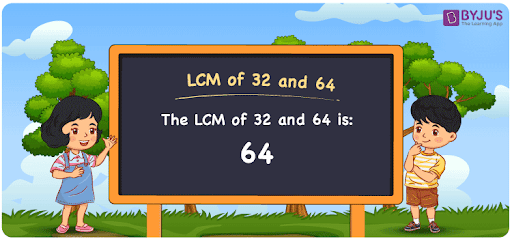# LCM of 32 and 64

LCM of 32 and 64 is 64. The Least Common Multiple or Lowest Common Multiple, known as LCM, is the least number that is divisible by the given lot of numbers. In the given set of numbers 32 and 64, 64 is the first(least or smallest) number that is common in the set of multiples of 32 and 64. There are other common multiples out of which 64 is the smallest. The LCM is also known as LCD, Least Common Divisor for 32 and 64 is 64. You can read more on LCM for further details.

## What is LCM of 32 and 64

The Least Common Multiple or Lowest Common Multiple of 32 and 64 is 64.## How to Find LCM of 32 and 64?

LCM of 32 and 64 can be determined using three methods:

• Prime Factorisation
• Division method
• Listing the multiples

### LCM of 32 and 64 Using Prime Factorisation Method

In the Prime Factorisation method, the numbers 32 and 64 can be expressed as;

32 = 2 × 2 × 2 × 2 × 2

64 = 2 × 2 × 2 × 2 × 2 × 2

Since 32 is the factor of 64, 32 is multiplied with the other factor of 64, which is 2 to get the LCM,

LCM (32, 64) = 2 × 2 × 2 × 2 × 2 × 2 = 64

### LCM of 32 and 64 Using Division Method

In the Division Method, the numbers 32 and 64 are divided together with prime divisors. Since 64 has the same factors of 32 also, 32 is divided with 2 to get the LCM.

Here 32 and 64 are divided as shown below;

 2 32 64 2 16 32 2 8 16 2 4 8 2 2 4 2 1 2 × 1 1

LCM (32, 64) = 2 × 2 × 2 × 2 × 2 × 3 × 3 = 64

### LCM of 32 and 64 Using Listing the Multiples

By listing all the multiples of 32 and 64, we can identify the LCM. Below is the list of multiples for 32 and 64

 Multiples of 32 Multiples of 64 32 64 64 128 96 192

LCM (32, 64) = 64

## Video Lesson on Applications of LCM## Solved Examples

What is the smallest number that is divisible by both 32 and 64?

Answer: 64 is the smallest number that is divisible by both 32 and 64.

What is the LCM for 2, 4, 8, 32 and 64?

Answer: LCM for 2, 4, 8, 32 and 64 is 64, as 2, 4, 8 are all factors of 32 and 64..

## Frequently Asked Questions on LCM of 32 and 64

### What is the LCM of 32 and 64?

The LCM of 32 and 64 is 64.

### Is the LCM of 32 and 64 the same as the Highest Common Factor of 32 and 64?

No. The Least Common Multiple of 32 and 64 is 64 and the Highest Common Factors of 32 and 64 is 32.

### What are the common factors of 32 and 64?

1, 2, 4, 8, 16 and 32 are the common factors of 32 and 64.

### What are the first 3 common multiples of 32 and 64?

The first three common multiples of 32 and 64 are 64, 128 and 192.

### Is the LCM of 4 and 32 same as the LCM of 4 and 64?

No. LCM of 4 and 32 is 32, whereas the LCM of 4 and 64 is 64. They are not the same.